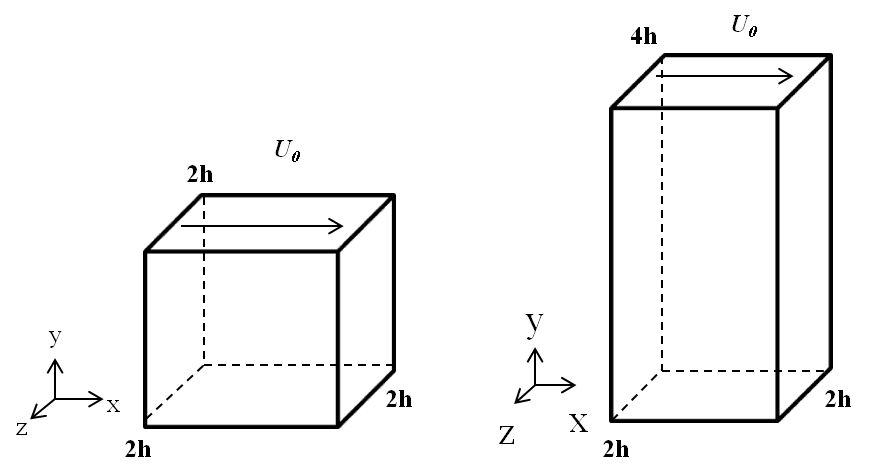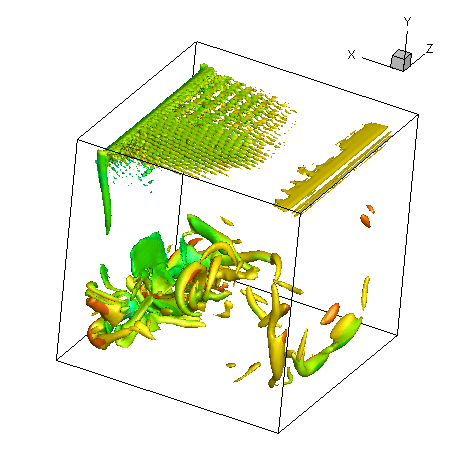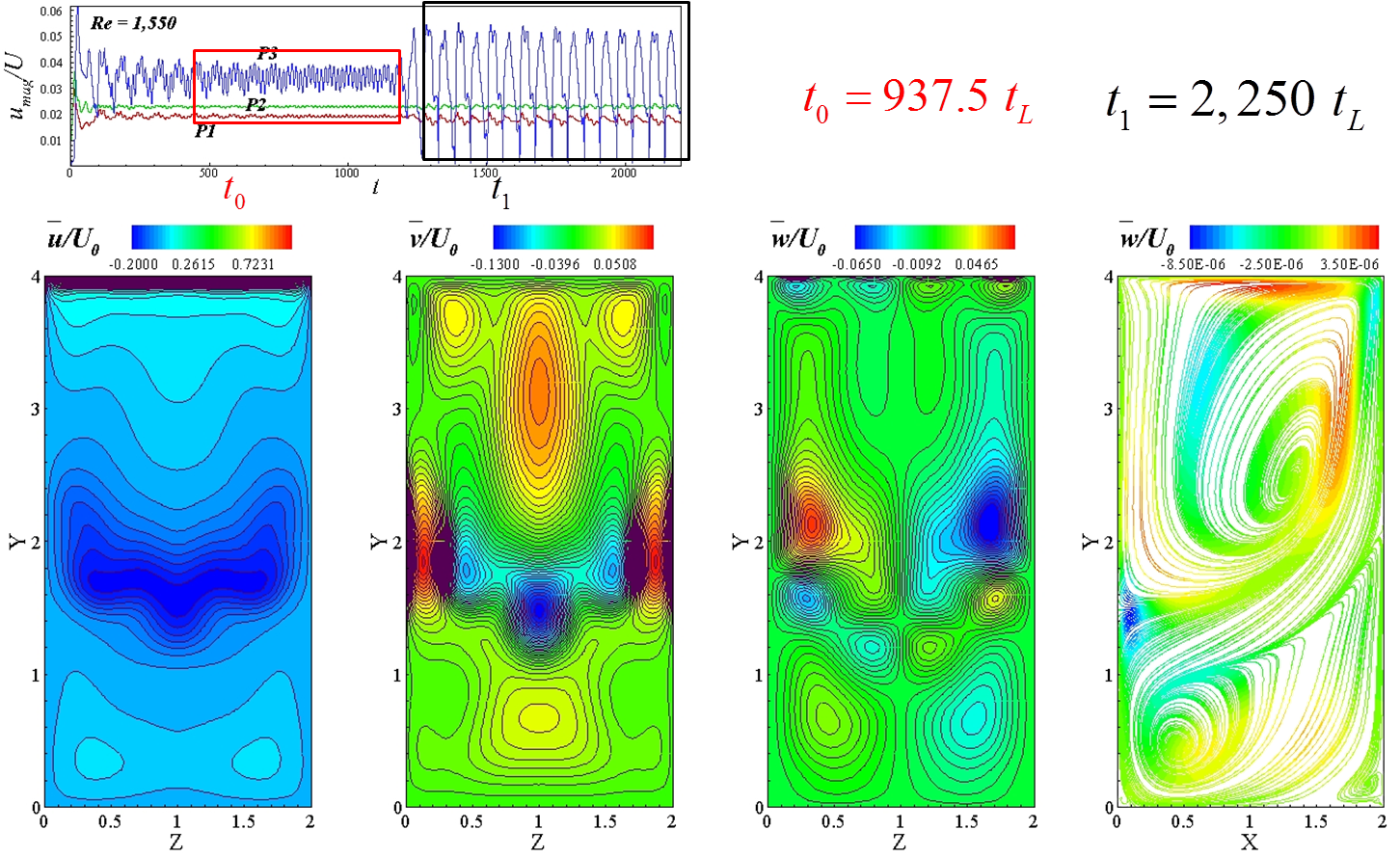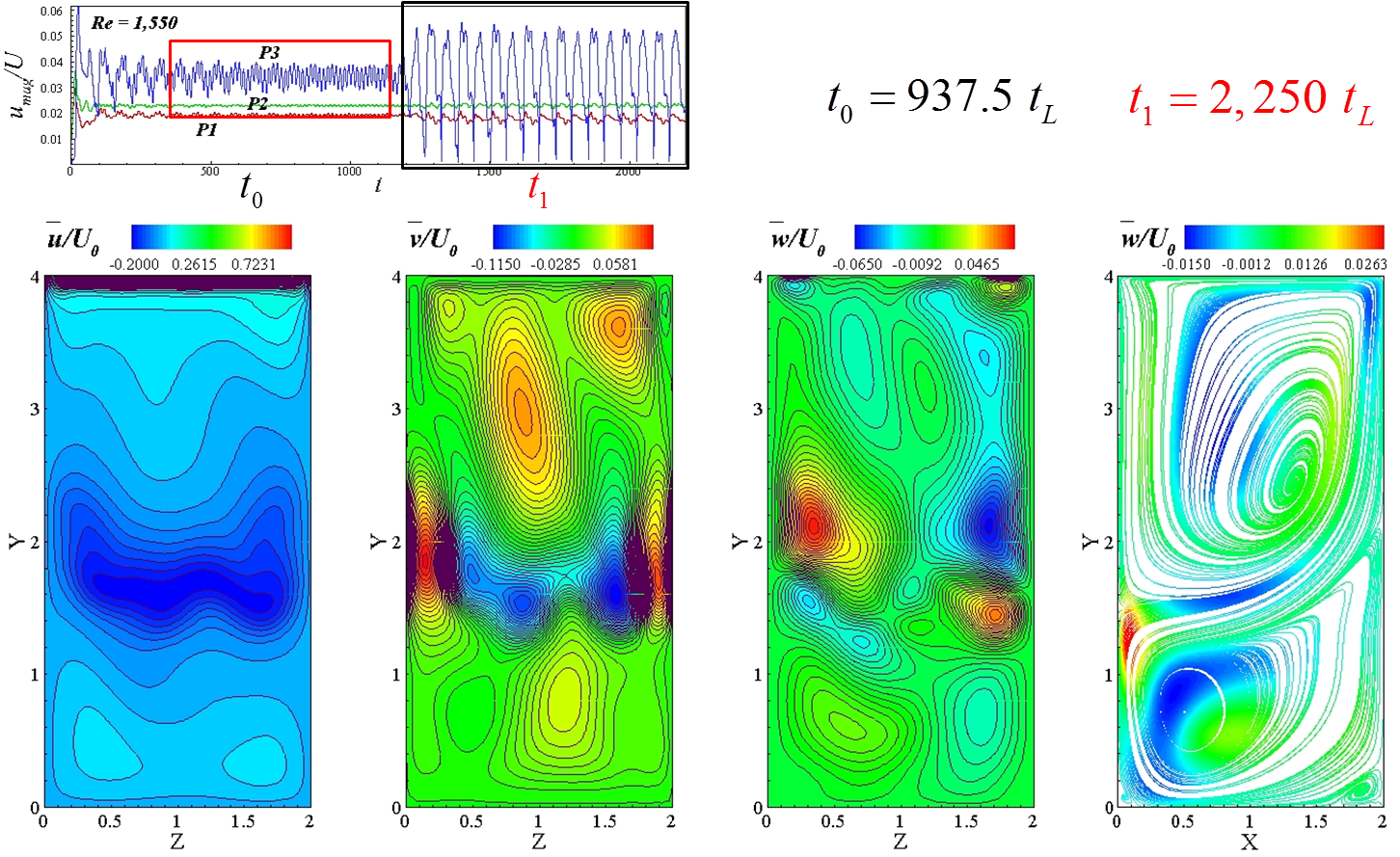# Computational Fluid Dynamics Laboratory

## Characterization of Oscillatory Instability in Lid Driven Cavity Flows using Lattice Boltzmann Method

The in-house LBM solver, namely LABLES, is enhanced to include large eddy simulation (LES) as turbulence modeling with two subgrid-scale (SGS) models and is applied for simulating flow in a three-dimensional lid driven cubic cavity and deep cavity with aspect ratio K=2.0. To ensure the correct implementation of the Bhatnagar-Gross-Krook (BGK) lattice Boltzmann equation (LBE), streaming process and boundary conditions, the LBM code is first validated for flow in the cubic cavity at Re=100, Re=400 and Re=1,000. To achieve a higher Reynolds number for cavity flows, SGS models including Smagorinsky model and Vreman model are used for the filtered BGK-LBE, considering the stability issues of the BGK collision operator. The code with SGS models is validated at Re=12,000. The effect of the D3Q19 and D3Q27 lattice models, optimal grid size and the performance of different SGS models are studied at Re=12,000.Under review for publication in Computers and Fluids (May 2013)Fig 1: The geometry and coordinate system of the lid driven cavities.Fig 2: isosurface of λ2 colored by V velocity magnitude at Re = 12,000 (80^3 grid; D3Q27 lattice model; Smagorinsky SGS model).

Based on the validation study in the cubic cavity, we apply the BGK-LBE with Smagorinsky model and D3Q27 lattice model on a 80^3 grid to the study the steady-oscillatory transition of the flows in lid driven cavities. For cubic cavity, oscillatory instabilities sets in via symmetry breaking about z=h plane at Re=2,250-2,300. The symmetry is recovered at Re=2,350, at which non-decaying oscillations exist in the flow field. For deep cavities with K=2, symmetry breaking is observed at Re=1,350-1,550, at which the flow first shows decaying oscillations and periodic oscillations occurs afer the flow is developed for a certain time.Fig 3: Instantaneous velocity U, V, W contours on X-plane and stremlines on Z-plane at t0; Oscillations mainly occur on the Z-plane.Fig 3: Instantaneous velocity U, V, W contours on X-plane and stremlines on Z-plane at t1; Oscillations transit to X-plane.

Prof. Frankel's MAIN PAGE